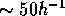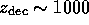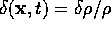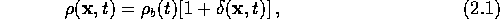Next: Linear Perturbation Theory Up: Origin of Structures Previous: Historical Perspective

## Gravitational Instability

According to the standard world picture, often referred to as the ``standard model'' (``Big Bang'' is somewhat misguiding), the Universe is approximately homogeneous on average over large enough scales. ``Large enough'' can be justified to be scales of size about 100Mpc (comparably, the size of the observable Universe is about 3000Mpc, in metric distance). The homogeneity requirement can also be applied very early in the history of the Universe, assuming an expanding Friedmann-Lemaître model. According to the gravitational instability theory, perturbations must have grown from small departures in this seemingly homogeneous mass distribution to the large-scales structures we observe today; such as galaxies, groups of galaxies, clusters, and even superclusters (Mpc). These density fluctuations where present at the decoupling of matter and radiation at redshift. Let us first take a look at how they grew from there.

The description of the gravitational instability theory can be found in numerous texts, e.g. Pad and P-80,P-93. I will follow the description of the latter two except where noted.

Perturbations of the density, or the density contrast, is given by. The mass density is thenwhereis the mean background mass density. Note that the spatial coordinatesare comoving; i.e. expanding with the model. One can imagine thatis the coordinates of a freely moving observer, t andbeing the time and the record of the density, respectively, kept by this observer.

Trond Hjorteland
Mon Jul 5 02:59:28 MET DST 1999